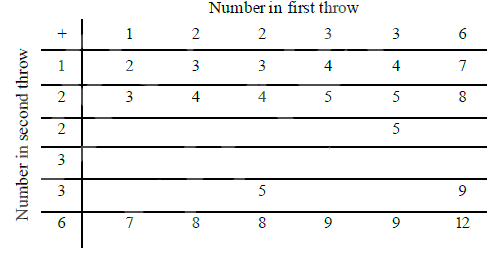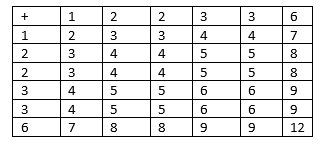# Class 10th Maths NCERT Solutions for Chapter 15 - Probability(Exercise 15.2)

## NCERT Solutions for Chapter 15 Exercise 15.2

In this page we have NCERT solutions for Class 10 Maths Chapter 15: probability for EXERCISE 15.2 on page 311 and 312 . Hope you like them and do not forget to like , social_share and comment at the end of the page.
Question 1.
Two customers Shyam and Ekta are visiting a particular shop in the same week (Tuesday to Saturday). Each is equally likely to visit the shop on any day as on another day. What is the probability that both will visit the shop on
(i) the same day?
(ii) consecutive days?
(iii) different days?
Solution
Total outcomes can be 25
(T,T) ,(T,W) ,(T,TH),(T,F),(T,S)
(W,T) ,(W,W) ,(W,TH),(W,F),(W,S)
(TH,T),(TH,W) ,(TH,TH),(TH,F),(TH,S)
(F,T) ,(F,W) ,(F,TH),(F,F),(F,S)
(S,T) ,(S,W) ,(S,TH),(S,F),(S,S)
Here T-Tuesday, W- Wednesday, TH=Thursday, F-Friday, S-Saturday
i. For Same day, Total number of favourable outcome are 5
(T,T),(W,W),(TH,TH),(F,F),(S,S)
So probability (P)
$P = \frac {5}{25} = \frac {1}{5}$

ii. for Consecutive days,, Total number of favourable outcome are 8
(T,W) ,(W,T) ,(W,TH),(TH,W) ,(TH,F),(F,TH),(F,S),(S,F)
So probability (P)
$P = \frac {8}{25}$

iii. For different days, Total number of favourable outcome =Total outcome- Number of days where came on same day= 25 -5 =20
So probability (P)
$P = \frac {20}{25}= \frac {4}{5}$

Question 2.
A die is numbered in such a way that its faces show the number 1, 2, 2, 3, 3, 6. It is thrown two times and the total score in two throws is noted. Complete the following table which gives a few values of the total score on the two throws:What is the probability that the total score is
(i) even
(ii) 6
(iii) at least 6
Solution
Complete tablei. Total score which are even can be 2,4,6,8,12
Total Favourable outcome = 18
$P(Even) = \frac {18}{36} = \frac {1}{2}$

ii. Total Favourable outcome = 4
$P(6) = \frac {4}{36} = \frac {1}{9}$

iii. Total score which are at least 6 can be 6,7,8,9,12
Total Favourable outcome = 15
$P(At \; least \; 6) = \frac {15}{36} = \frac {5}{12}$

Question 3.
A bag contains 5 red balls and some blue balls. If the probability of drawing a blue ball is doubles that of a red ball, determine the number of blue balls in the bag.?
Solution
Let x be the number of blue balls in the bag.Then total number of balls=5+x
$P(Blue) = \frac {x}{x+ 5}$
$P(Red) = \frac {5}{x+ 5}$
Now as per question
$P(Blue) = 2 \times P(Red)$
$\frac {x}{x+ 5} = 2 \times \frac {5}{x+ 5}$
Solving this
x=10

Question 4.
A box contains 12 balls out of which x are black. If one ball is drawn at random from the box, what is the probability that it will be a black ball? If 6 more black balls are put in the box, the probability of drawing a black ball is now double of what it was before. Find x.
Solution
Total Number of balls=12
Number of Black ball=x
$P(black) = \frac {x}{12}$

Total number of ball after the addition of 6 balls = 12+6=18
Number of black ball after addition= x+6
$P(black \; after \; addition) = \frac {x+6}{18}$
Now as per question
$P(black \; after \; addition)= 2 P(black)$
$\frac {x+6}{18} = 2 \times \frac {x}{12}$
Solving this
x=3

Question 5.
A jar contains 24 marbles, some are green and others are blue. If a marble is drawn at random from the jar, the probability that it is green is 2/3. Find the number of blue balls in the jar.
Solution
Let x be the blue balls
Then number of green balls = 24 -x
Now
P(Green) = \frac {24 -x}{24}$Now as per question$\frac {24 -x}{24} = \frac {2}{3}$Solving this x=8 link to this page by copying the following text Also ReadGo back to Class 10 Main Page using below links ### Practice Question Question 1 What is$1 - \sqrt {3}\$ ?
A) Non terminating repeating
B) Non terminating non repeating
C) Terminating
D) None of the above
Question 2 The volume of the largest right circular cone that can be cut out from a cube of edge 4.2 cm is?
A) 19.4 cm3
B) 12 cm3
C) 78.6 cm3
D) 58.2 cm3
Question 3 The sum of the first three terms of an AP is 33. If the product of the first and the third term exceeds the second term by 29, the AP is ?
A) 2 ,21,11
B) 1,10,19
C) -1 ,8,17
D) 2 ,11,20